# Summarize and Explore the Data

## Intro

The document introduces the SmartEDA package and how it can help you to build exploratory data analysis.

SmartEDA includes multiple custom functions to perform initial exploratory analysis on any input data describing the structure and the relationships present in the data. The generated output can be obtained in both summary and graphical form. The graphical form or charts can also be exported as reports.

सर्वस्य लोचनं शास्त्रं
Science is the only eye

अनेकसंशयोच्छेदि, परोक्षार्थस्य दर्शक|
सर्वस्य लोचनं शास्त्रं, यस्य नास्त्यन्ध एव सः ||

It blasts many doubts, foresees what is not obvious |
Science is the eye of everyone, one who hasnt got it, is like a blind ||

SmartEDA package helps you to construct a good base of data understanding. The capabilities and functionalities are listed below

1. SmartEDA package will make you capable of applying different types of EDA without having to
• remember the different R package names
• write lengthy R scripts
• manual effort to prepare the EDA report
2. No need to categorize the variables into Character, Numeric, Factor etc. SmartEDA functions automatically categorize all the features into the right data type (Character, Numeric, Factor etc.) based on the input data.

3. ggplot2 functions are used for graphical presentation of data

4. Rmarkdown and knitr functions were used for build HTML reports

To summarize, SmartEDA package helps in getting the complete exploratory data analysis just by running the function instead of writing lengthy r code.

## Data

In this vignette, we will be using a simulated data set containing sales of child car seats at 400 different stores.

Data Source ISLR package.

Install the package “ISLR” to get the example data set.

install.packages("ISLR")
library("ISLR")
install.packages("SmartEDA")
library("SmartEDA")
## Load sample dataset from ISLR pacakge
Carseats= ISLR::Carseats

### Overview of the data

Understanding the dimensions of the dataset, variable names, overall missing summary and data types of each variables

# Overview of the data - Type = 1
ExpData(data=Carseats,type=1)

# Structure of the data - Type = 2
ExpData(data=Carseats,type=2)
• Overview of the data
Descriptions Obs
Sample size (Nrow) 400
No. of Variables (Ncol) 11
No. of Numeric Variables 8
No. of Factor Variables 3
No. of Text Variables 0
No. of Logical Variables 0
No. of Date Variables 0
No. of Zero variance Variables (Uniform) 0
%. of Variables having complete cases 100% (11)
%. of Variables having <50% missing cases 0% (0)
%. of Variables having >50% missing cases 0% (0)
%. of Variables having >90% missing cases 0% (0)
• Structure of the data
S.no Variable Name Variable Type % of Missing No. of Unique values
1 Sales numeric 0 336
2 CompPrice numeric 0 73
3 Income numeric 0 98
5 Population numeric 0 275
6 Price numeric 0 101
7 ShelveLoc* factor 0 3
8 Age numeric 0 56
9 Education numeric 0 9
10 Urban* factor 0 2
11 US* factor 0 2

## Exploratory data analysis (EDA)

This function shows the EDA output for 3 different cases

1. Target variable is not defined
2. Target variable is continuous
3. Target variable is categorical

### Example for case 1: Target variable is not defined

#### 1.1 Summary of numerical variables

Summary of all numerical variables

ExpNumStat(Carseats,by="A",gp=NULL,Qnt=seq(0,1,0.1),MesofShape=2,Outlier=TRUE,round=2,Nlim=10)

#### 1.2 Distributions of numerical variables

Graphical representation of all numeric features

• Density plot (Univariate)
# Note: Variable excluded (if unique value of variable which is less than or eaual to 10 [nlim=10])
plot1 <- ExpNumViz(Carseats,gp=NULL,nlim=10,Page=c(2,2),sample=8)
plot1[]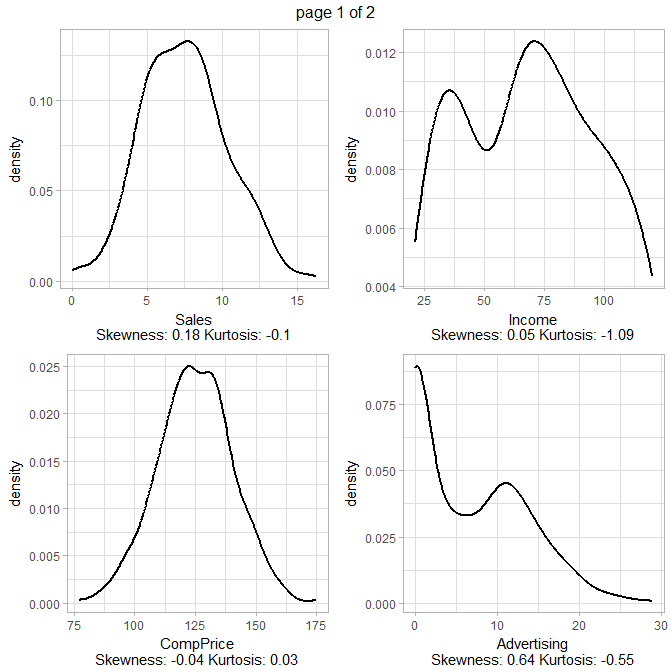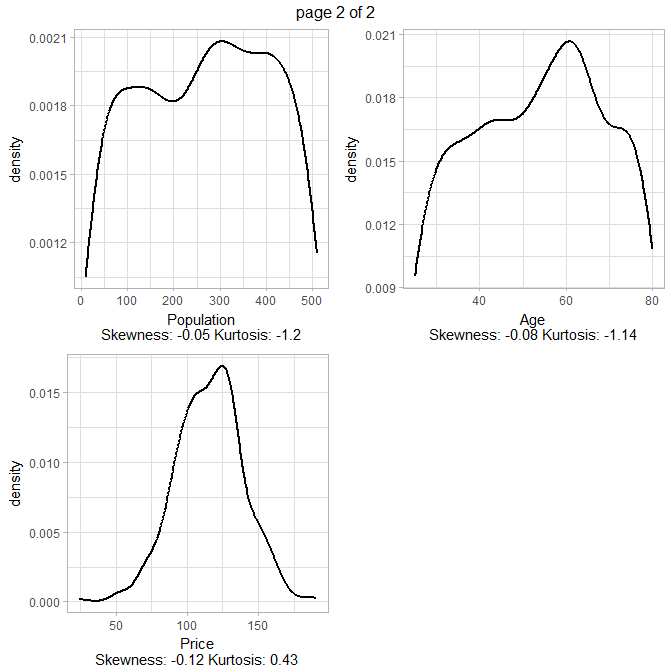#### 1.3. Summary of categorical variables

• frequency for all categorical independent variables
ExpCTable(Carseats,Target=NULL,margin=1,clim=10,nlim=NULL,round=2,bin=NULL,per=T)
Variable Valid Frequency Percent CumPercent
ShelveLoc Good 85 21.25 45.25
ShelveLoc Medium 219 54.75 100.00
ShelveLoc TOTAL 400 NA NA
Urban No 118 29.50 29.50
Urban Yes 282 70.50 100.00
Urban TOTAL 400 NA NA
US No 142 35.50 35.50
US Yes 258 64.50 100.00
US TOTAL 400 NA NA

NA is Not Applicable

#### 1.4. Distributions of categorical variables

• Bar plots for all categorical variables
plot2 <- ExpCatViz(Carseats,gp=NULL,fname=NULL,clim=10,margin=2,Page = c(2,1),sample=4)
plot2[]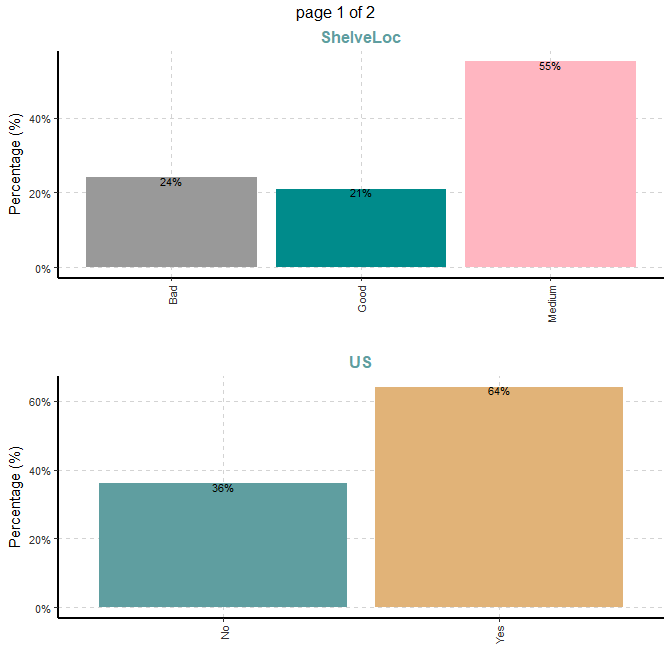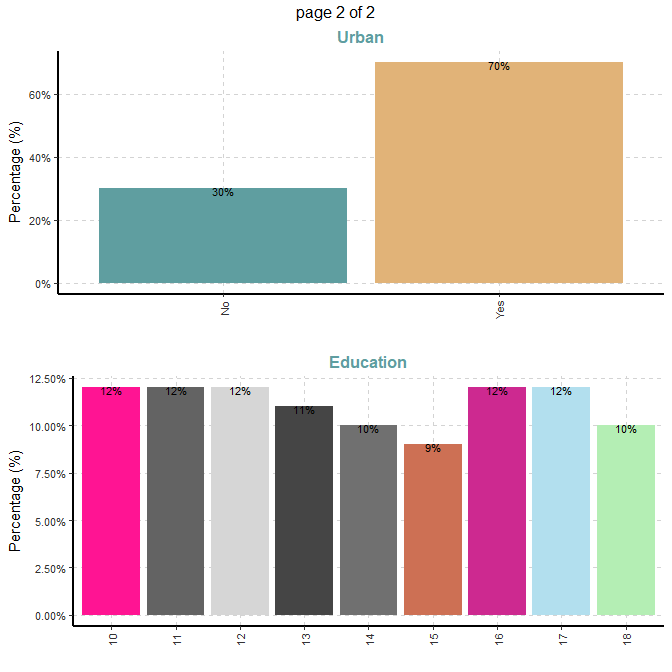### Example for case 2: Target variable is continuous

#### 2.1. Target variable

Summary of continuous dependent variable

1. Variable name - Price
2. Variable description - Price company charges for car seats at each site
summary(Carseats[,"Price"])
##      Price
##  Min.   : 24.0
##  1st Qu.:100.0
##  Median :117.0
##  Mean   :115.8
##  3rd Qu.:131.0
##  Max.   :191.0

#### 2.2 Summary of numerical variables

Summary statistics when dependent variable is continuous Price.

ExpNumStat(Carseats,by="A",gp="Price",Qnt=seq(0,1,0.1),MesofShape=1,Outlier=TRUE,round=2)

If Target variable is continuous, summary statistics will add the correlation column (Correlation between Target variable vs all independet variables)

#### 2.3 Distributions of numerical variables

Graphical representation of all numeric variables

• Scatter plot (Bivariate)

Scatter plot between all numeric variables and target variable Price. This plot help to examine how well a target variable is correlated with dependent variables.

Dependent variable is Price (continuous).

#Note: sample=8 means randomly selected 8 scatter plots
#Note: nlim=4 means included numeric variable with unique value is more than 4
plot3 <- ExpNumViz(Carseats,gp="Price",nlim=4,fname=NULL,col=NULL,Page=c(2,2),sample=8)
plot3[]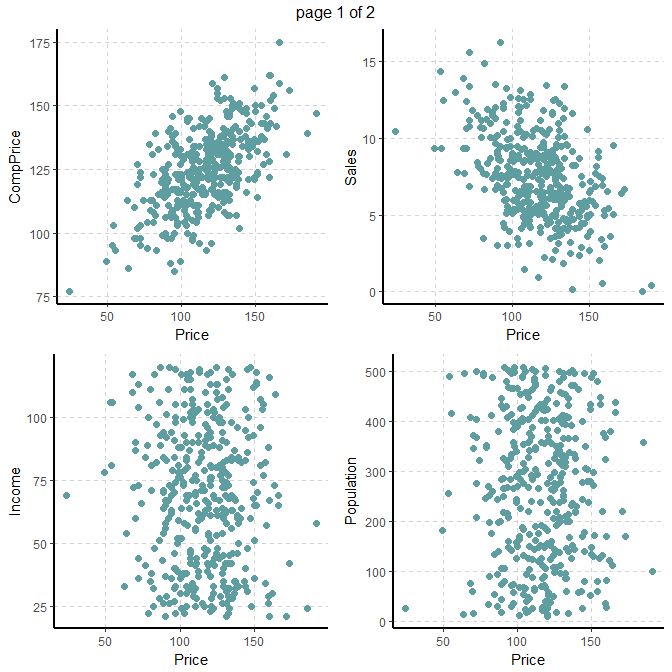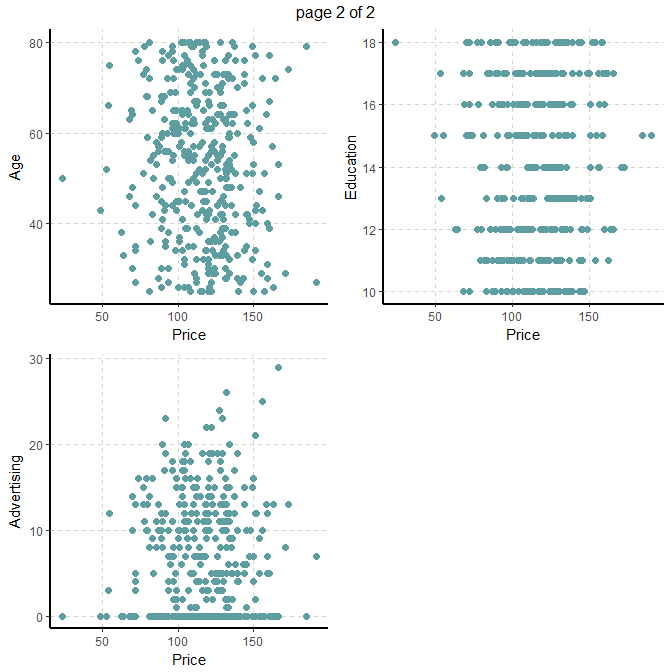#### 2.4. Summary of categorical variables

Summary of categorical variables

• frequency for all categorical independent variables by descretized Price
##bin=4, descretized 4 categories based on quantiles
ExpCTable(Carseats,Target="Price",margin=1,clim=10,nlim=NULL,round=2,bin=4,per=F)

### Example for case 3: Target variable is categorical

#### 3.1. Target variable

Summary of categorical dependent variable

1. Variable name - Urban
2. Variable description - Whether the store is in an urban or rural location
Urban Frequency Descriptions
No 118 Store location
Yes 282 Store location

#### 3.1 Summary of numerical variables

Summary of all numeric variables

ExpNumStat(Carseats,by="GA",gp="Urban",Qnt=seq(0,1,0.1),MesofShape=2,Outlier=TRUE,round=2)

#### 3.2 Distributions of Numerical variables

• Box plots for all numerical variables vs categorical dependent variable - Bivariate comparision only with categories

Boxplot for all the numeric attributes by each category of Urban

plot4 <- ExpNumViz(Carseats,gp="Urban",type=1,nlim=NULL,fname=NULL,col=c("pink","yellow","orange"),Page=c(2,2),sample=8)
plot4[]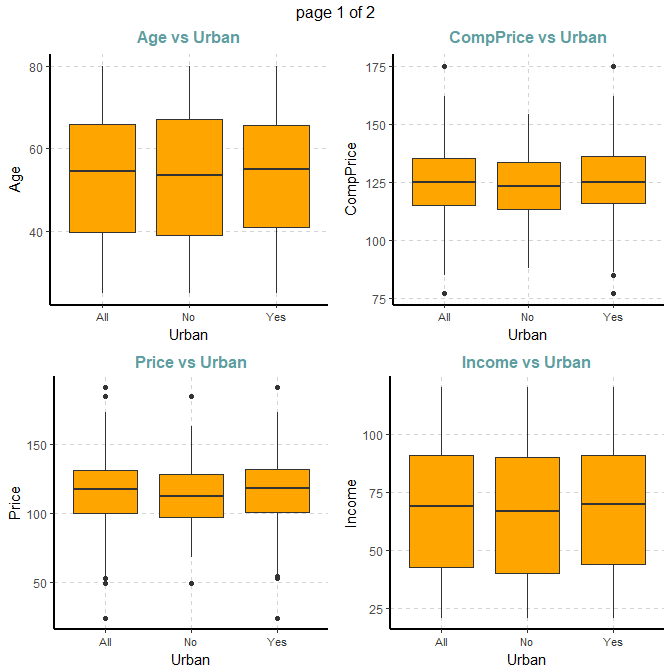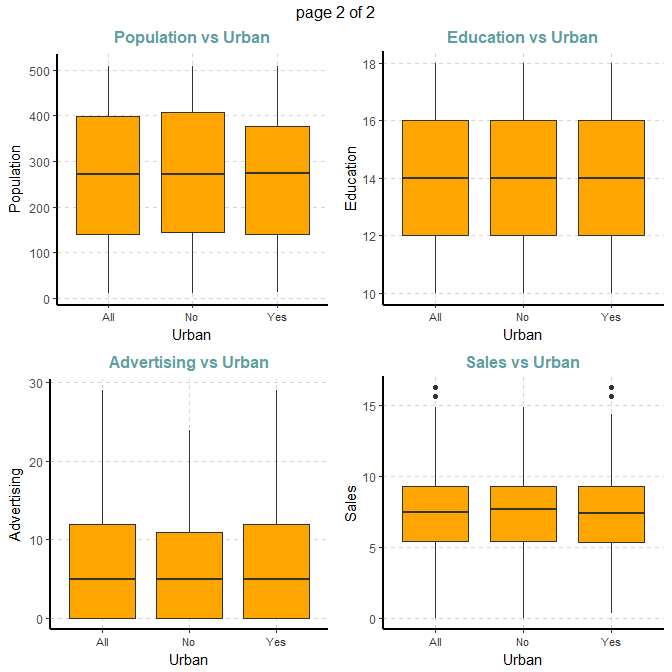#### 3.3 Summary of categorical variables

Cross tabulation with target variable

• Custom tables between all categorical independent variables and target variable Urban
ExpCTable(Carseats,Target="Urban",margin=1,clim=10,nlim=NULL,round=2,bin=NULL,per=F)
VARIABLE CATEGORY Urban:No Urban:Yes TOTAL
ShelveLoc Good 28 57 85
ShelveLoc Medium 68 151 219
ShelveLoc TOTAL 118 282 400
US No 46 96 142
US Yes 72 186 258
US TOTAL 118 282 400

Information Value

ExpCatStat(Carseats,Target="Urban",Label="Store Location",result = "IV",clim=10,nlim=5,Pclass="Yes")
Variable Target Class Out_1 Out_0 TOTAL Per_1 Per_0 Odds WOE IV Ref_1 Ref_0
ShelveLoc Urban Bad 74 22 96 0.262 0.186 1.409 0.343 0.026 Yes No
ShelveLoc Urban Good 57 28 85 0.202 0.237 0.852 -0.160 0.006 Yes No
ShelveLoc Urban Medium 151 68 219 0.535 0.576 0.929 -0.074 0.003 Yes No
US Urban No 96 46 142 0.340 0.390 0.872 -0.137 0.007 Yes No
US Urban Yes 186 72 258 0.660 0.610 1.082 0.079 0.004 Yes No

Statistical test

ExpCatStat(Carseats,Target="Urban",Label="Store Location",result = "Stat",clim=10,nlim=5,Pclass="Yes")
Variable Target Unique Chi-squared p-value df IV Value Cramers V Degree of Association Predictive Power
ShelveLoc Urban 3 2.738 0.254 2 0.035 0.08 Very Weak Not Predictive
US Urban 2 0.684 0.408 1 0.011 0.04 Very Weak Not Predictive

#### 3.4. Distributions of categorical variables

Stacked bar plot with vertical or horizontal bars for all categorical variables

plot5 <- ExpCatViz(Carseats,gp="Urban",fname=NULL,clim=10,col=NULL,margin=2,Page = c(2,1),sample=2)
plot5[]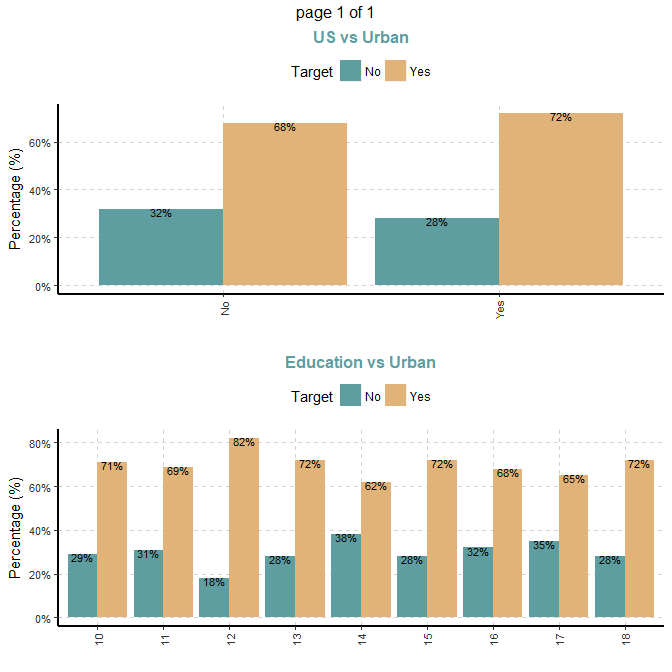### Quantile-quantile plot for numeric variables

Function definition:

ExpOutQQ (data,nlim=NULL,fname=NULL,Page=NULL,sample=NULL)
data    : Input dataframe or data.table
nlim    : numeric variable limit
fname   : output file name (Output will be in PDF format)
Page    : output pattern. if Page=c(3,2), It will generate 6 plots with 3 rows and 2 columns
sample  : random number of plots

Carseats data from ISLR package:

options(width = 150)
CData = ISLR::Carseats
qqp <- ExpOutQQ(CData,nlim=10,fname=NULL,Page=c(2,2),sample=4)
qqp[]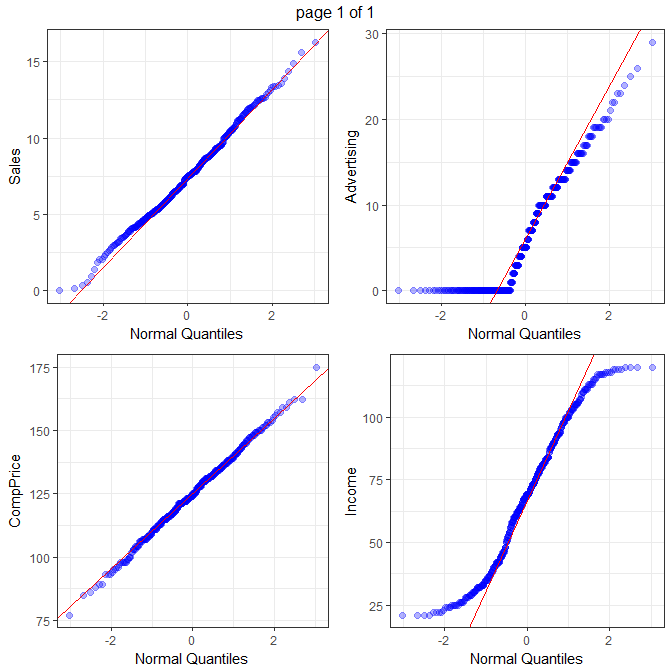## Parallel Co-ordinate plots

Function definition:

ExpParcoord (data,Group=NULL,Stsize=NULL,Nvar=NULL,Cvar=NULL,scale=NULL)
data    : Input dataframe or data.table
Group   : stratification variables
Stsize  : vector of startum sample sizes
Nvar    : vector of numerice variables, default it will consider all the numeric variable from data
Cvar    : vector of categorical variables, default it will consider all the categorical variable
scale   : scale the variables in the parallel coordinate plot[Default normailized with minimum of the variable is zero and maximum of the variable is one]

#### Defualt ExpParcoord funciton

ExpParcoord(CData,Group=NULL,Stsize=NULL,Nvar=c("Price","Income","Advertising","Population","Age","Education"))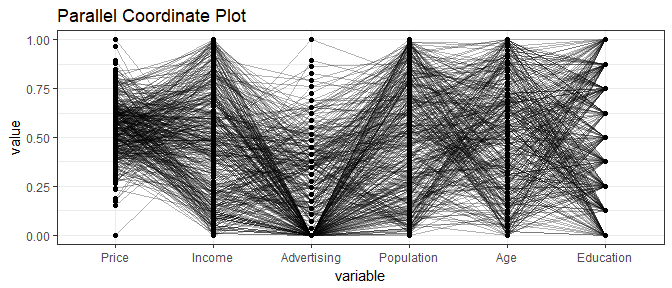#### With Stratified rows and selected columns only

ExpParcoord(CData,Group="ShelveLoc",Stsize=c(10,15,20),Nvar=c("Price","Income"),Cvar=c("Urban","US"))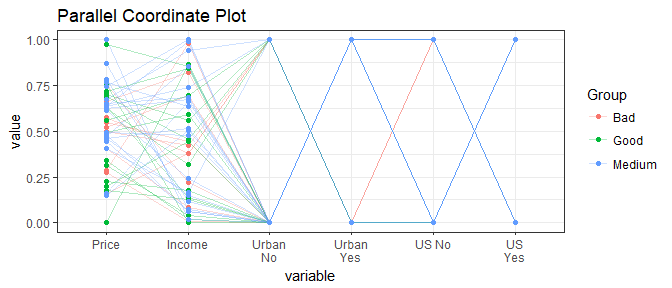#### Without stratification

ExpParcoord(CData,Group="ShelveLoc",Nvar=c("Price","Income"),Cvar=c("Urban","US"),scale=NULL)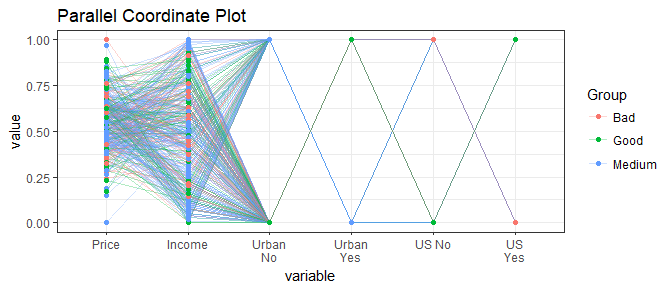#### Scale changed std: univariately, subtract mean and divide by standard deviation

ExpParcoord(CData,Group="US",Nvar=c("Price","Income"),Cvar=c("ShelveLoc"),scale="std")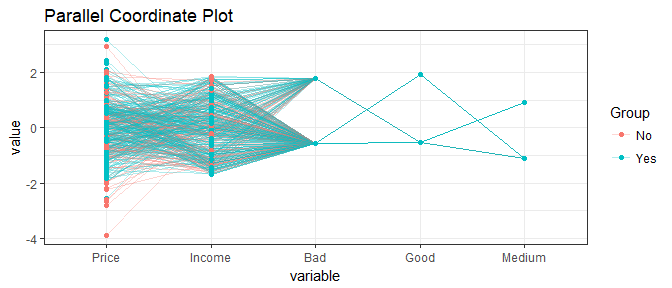#### Selected numeric variables

ExpParcoord(CData,Group="ShelveLoc",Stsize=c(10,15,20),Nvar=c("Price","Income","Advertising","Population","Age","Education"))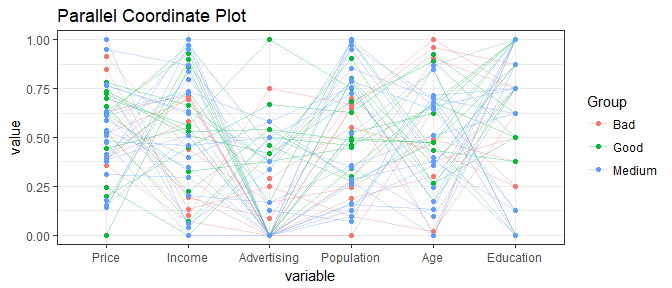#### Selected categorical variables

ExpParcoord(CData,Group="US",Stsize=c(15,50),Cvar=c("ShelveLoc","Urban"))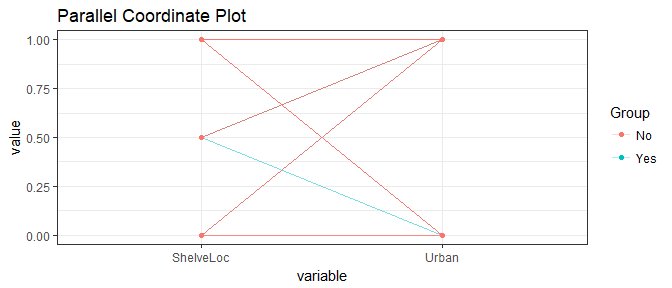## Customized Summary Statistics

Used ‘data.table’ package functions

Function definition:

ExpCustomStat(data,Cvar=NULL,Nvar=NULL,stat=NULL,gpby=TRUE,filt=NULL,dcast=FALSE)

ExpCustomStat examples

ExpCustomStat(Carseats,Cvar="Urban",Nvar=c("Age","Price"),stat=c("mean","count"),gpby=TRUE,dcast=F)
Urban Attribute mean count
Yes Age 53.62057 282
No Age 52.61017 118
Yes Price 116.51418 282
No Price 114.07627 118
ExpCustomStat(Carseats,Cvar="Urban",Nvar=c("Age","Price"),stat=c("mean","count"),gpby=TRUE,dcast=T)
Attribute mean_No mean_Yes count_No count_Yes
Age 52.61017 53.62057 118 282
Price 114.07627 116.51418 118 282
ExpCustomStat(Carseats,Cvar=c("Urban","ShelveLoc"),Nvar=c("Age","Price","Advertising","Sales"),stat=c("mean"),gpby=FALSE,dcast=T)
Attribute ShelveLoc_Bad ShelveLoc_Good ShelveLoc_Medium Urban_No Urban_Yes
Advertising 6.218750 7.352941 6.538813 6.203390 6.815603
Age 52.052083 52.611765 54.155251 52.610169 53.620567
Price 114.270833 117.882353 115.652968 114.076271 116.514184
Sales 5.522917 10.214000 7.306575 7.563559 7.468191